### Iterative Methods: SOR Method

#### The Successive Over-Relaxation (SOR) Method

The successive over-relaxation (SOR) method is another form of the Gauss-Seidel method in which the new estimate at iteration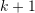for the component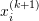is calculated as the weighted average of the previous estimate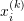and the estimate using Gauss-Seidel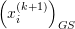: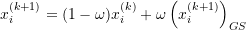wherecan be obtained using Equation 1 in the Gauss-Seidel method. The weight factoris chosen such that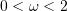. If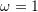, then the method is exactly the Gauss-Seidel method. Otherwise, to understand the effect ofwe will rearrange the above equation to: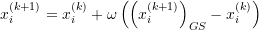If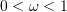, then, the method gives a new estimate that lies somewhere between the old estimate and the Gauss-Seidel estimate, in this case, the algorithm is termed: “under-relaxation” (Figure 1). Under-relaxation can be used to help establish convergence if the method is diverging. If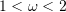, then the new estimate lies on the extension of the vector joining the old estimate and the Gauss-Seidel estimate and hence the algorithm is termed: “over-relaxation” (Figure 1). Over-relaxation can be used to speed up convergence of a slow-converging process. The following code defines and uses the SOR procedure to calculate the new estimates. Compare with the Gauss-Seidel procedure shown above.

View Mathematica Code
SOR[A_, b_, x_, w_] := (n = Length[A];
xnew = Table[0, {i, 1, n}];
Do[xnew[[i]] = (1 - w)*x[[i]] + w*(b[[i]] - Sum[A[[i, j]] x[[j]], {j, i + 1, n}] - Sum[A[[i, j]] xnew[[j]], {j, 1, i - 1}])/A[[i, i]], {i, 1,  n}];
xnew)
A = {{3, -0.1, -0.2}, {0.1, 7, -0.3}, {0.3, -0.2, 10}}
b = {7.85, -19.3, 71.4}
x = {{1, 1, 1.}};
MaxIter = 100;
ErrorTable = {1};
eps = 0.001;
i = 2;
omega=1.1;

While[And[i <= MaxIter, Abs[ErrorTable[[i - 1]]] > eps],
xi = SOR[A, b, x[[i - 1]],omega]; x = Append[x, xi]; ei = Norm[x[[i]] - x[[i - 1]]]/Norm[x[[i]]];
ErrorTable = Append[ErrorTable, ei];
i++]
x // MatrixForm
ErrorTable // MatrixForm

View Python Code
import numpy as np
def SOR(A, b, x, w):
A = np.array(A); b = np.array(b); x = np.array(x);
n = len(A)
xnew = np.zeros(n)
for i in range(n):
xnew[i] = (1 - w)*x[i] + w*(b[i] - sum([A[i, j]*x[j] for j in range(i + 1, n)]) - sum([A[i, j]*xnew[j] for j in range(i)]))/A[i, i]
return xnew

A = [[3, -0.1, -0.2], [0.1, 7, -0.3], [0.3, -0.2, 10]]
b = [7.85, -19.3, 71.4]
x = [[1, 1, 1.]]
MaxIter = 100
ErrorTable = 
eps = 0.001
i = 1
omega = 1.1

while i <= MaxIter and abs(ErrorTable[i - 1]) > eps:
xi = SOR(A, b, x[i - 1],omega)
x.append(xi)
ei = np.linalg.norm(x[i] - x[i - 1])/np.linalg.norm(x[i])
ErrorTable.append(ei)
i+=1
print("x:",np.array(x))
print("ErrorTable:",np.vstack(ErrorTable))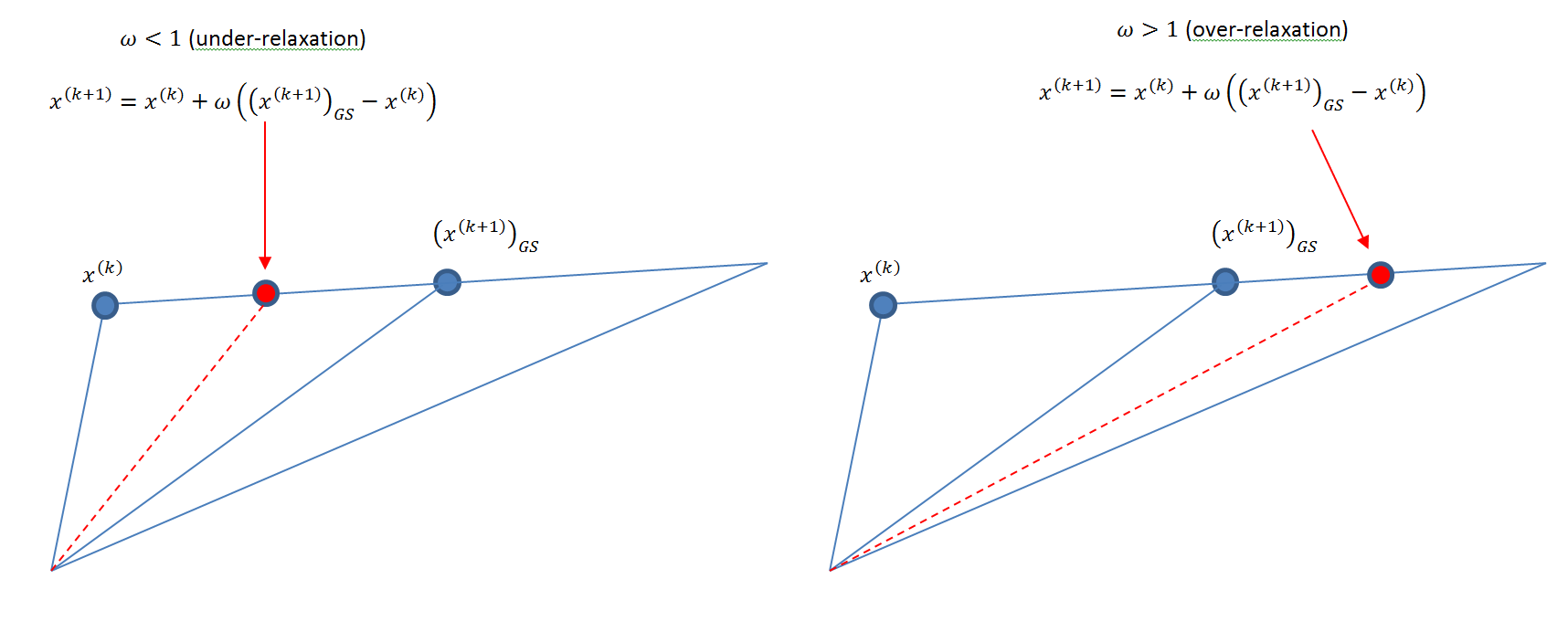Figure 1. Illustration of under- and over-relaxation methods

### Lecture Video

The following video covers the SOR method.